# electromagnetic fields theory If a load with a mass of m Ig is placed on the loop as shown in Figure 2, investigate...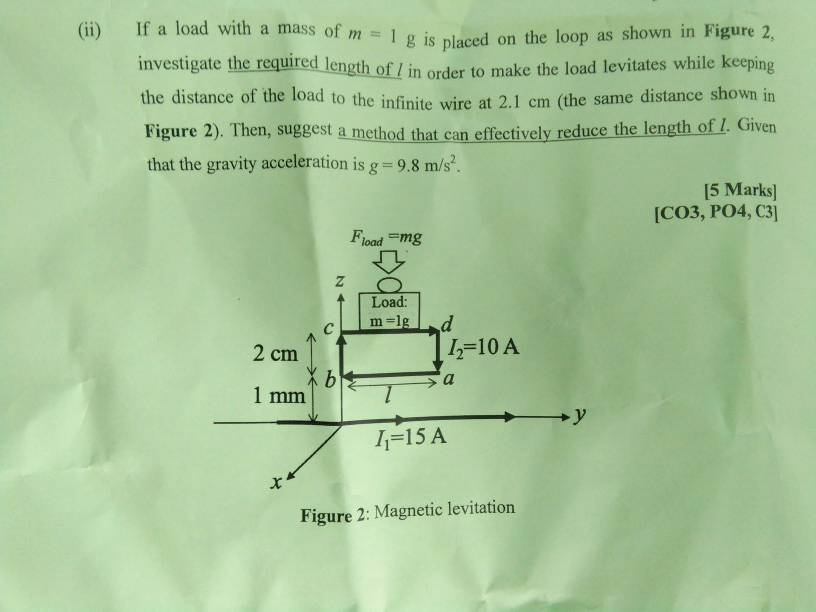electromagnetic fields theory

If a load with a mass of m Ig is placed on the loop as shown in Figure 2, investigate the required length of 1 in order to make the load levitates while keeping (ii) the distance of the load to the infinite wire at 2.1 cm distance shown in (the same Figure 2). Then, suggest a method that can effectively reduce the length of l. Given that the gravity acceleration is g 9.8 m/s2. 15 Marks] [CO3, PO4, C3] Fload mg Z Load: m-1g d =10 A C 2 cm X b 1 mm a +y -15 A Figure 2: Magnetic levitation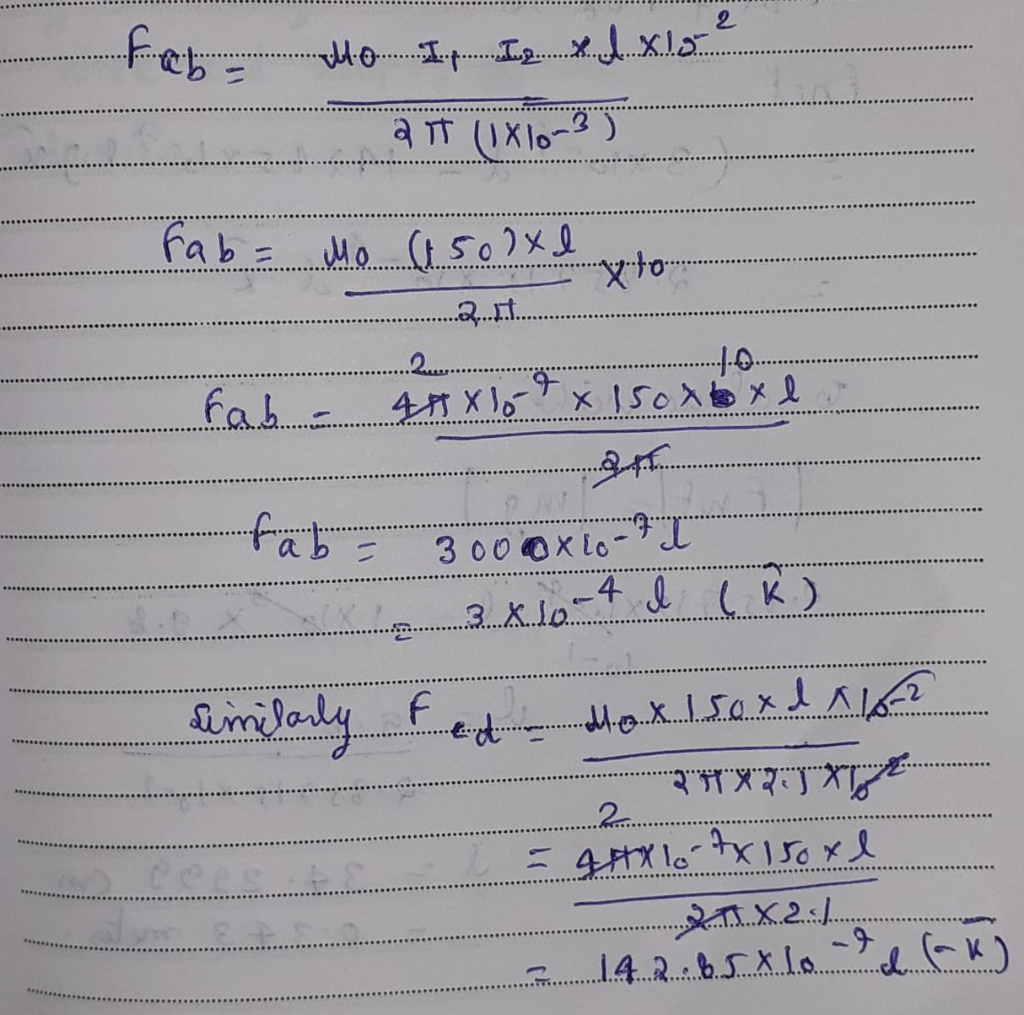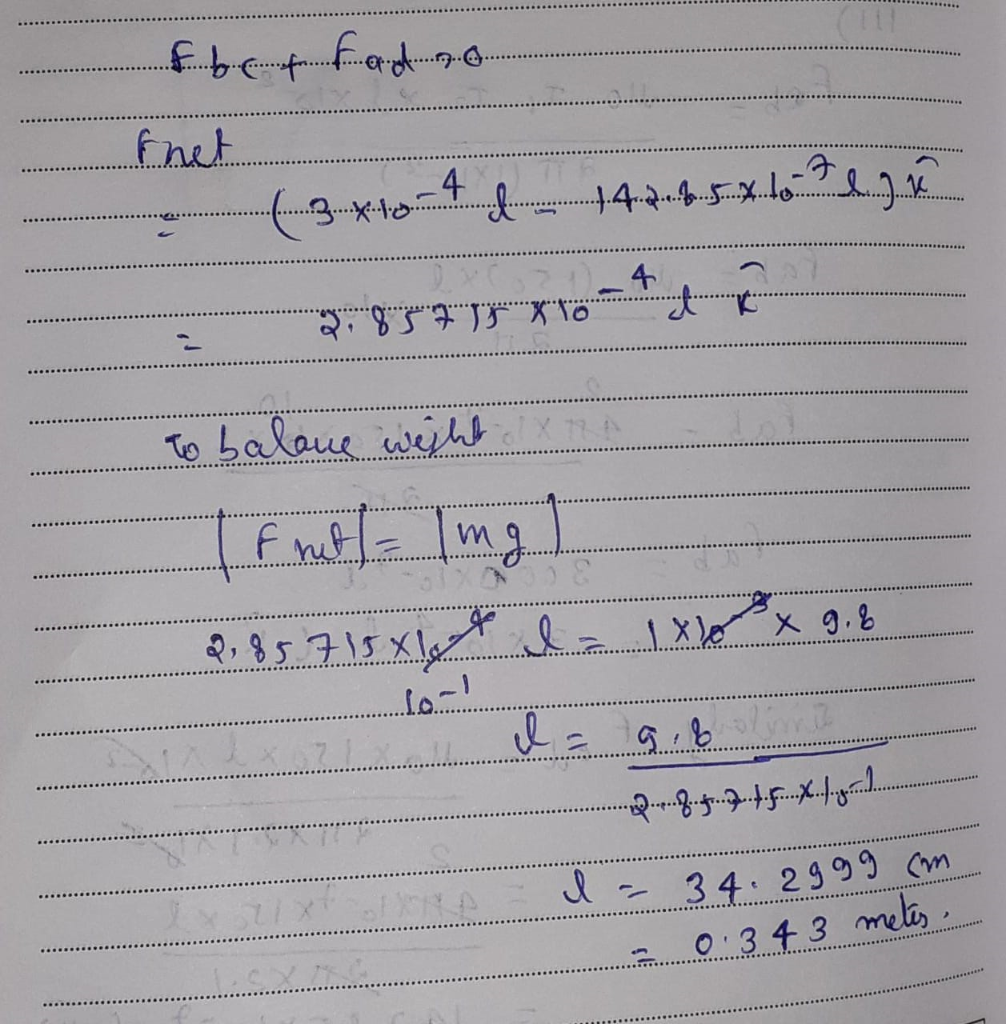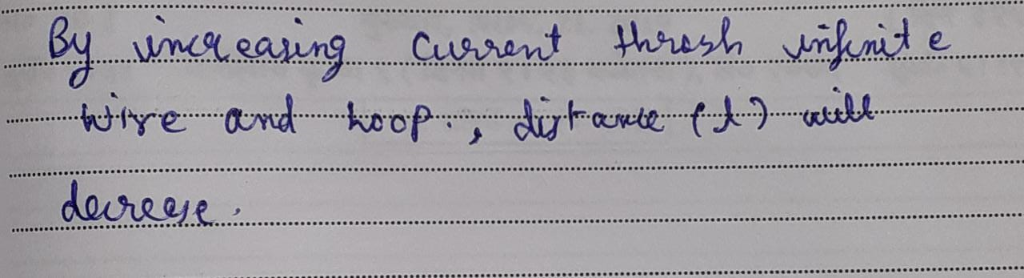##### Add Answer of: electromagnetic fields theory If a load with a mass of m Ig is placed on the loop as shown in Figure 2, investigate...
Similar Homework Help Questions
• ### (4). (8 pts). A soil profile is shown in below Figure. A uniformly distributed load Ao is applied at the surface of soil. Given the preconsolidation pressure is between swell index and compressio...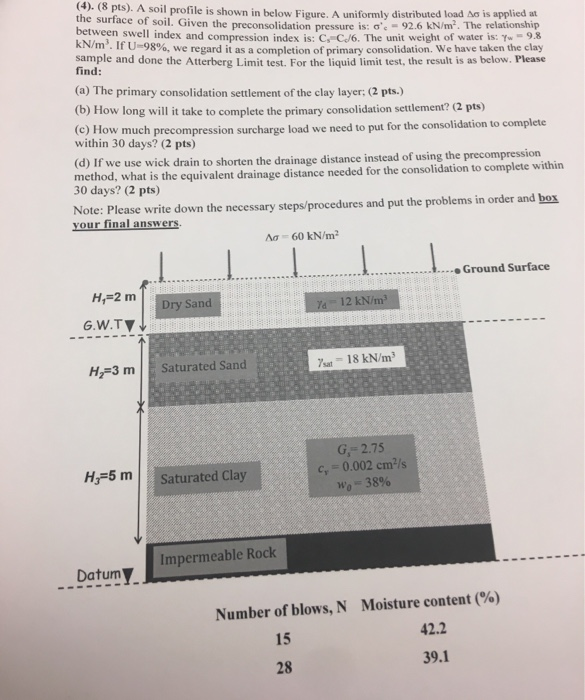(4). (8 pts). A soil profile is shown in below Figure. A uniformly distributed load Ao is applied at the surface of soil. Given the preconsolidation pressure is between swell index and compression index is: C C/6. The unit weight of water is: -9.38 kN/m. If U-98% we regard it as a completion of primary consolidation. We have taken the clay sample and done the Atterberg Limit test. For the liquid limit test, the result is as below. Please ơ'-926...

• ### 3. In Figure 2, what is the direction of the current induced in the resistor at...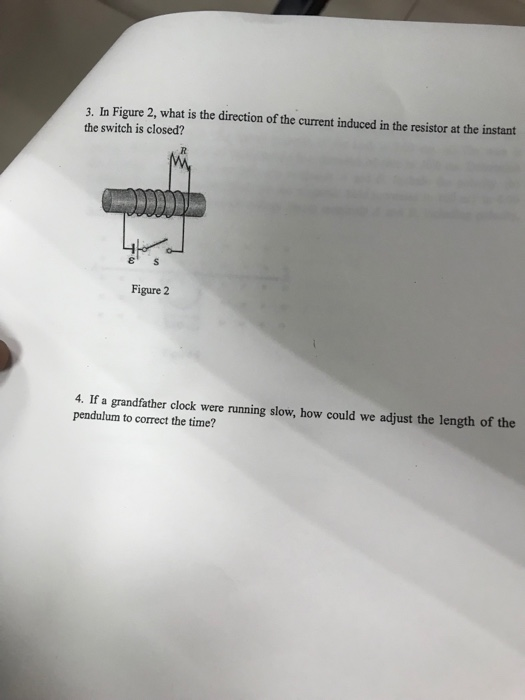3. In Figure 2, what is the direction of the current induced in the resistor at the instant the switch is closed? ㄐㄧ - Figure 2 4. If a grandfather clock were running slow, how could we adjust the length of the pendulum to correct the time? 4. A 2.00 C charged 1.00 g cork ball is suspended vertically on a 0.500 m long light string in the presence of a uniform downward-directed electric field of magnitude E-1.00x10 N/C. If...

• ### This question is worth 20 marks Figure 2 shows a decorator's plank, which is 5 m in length, supported on two trestles. The mass of the plank is 37 kg and the mass of the decorator is 70 kg. In or...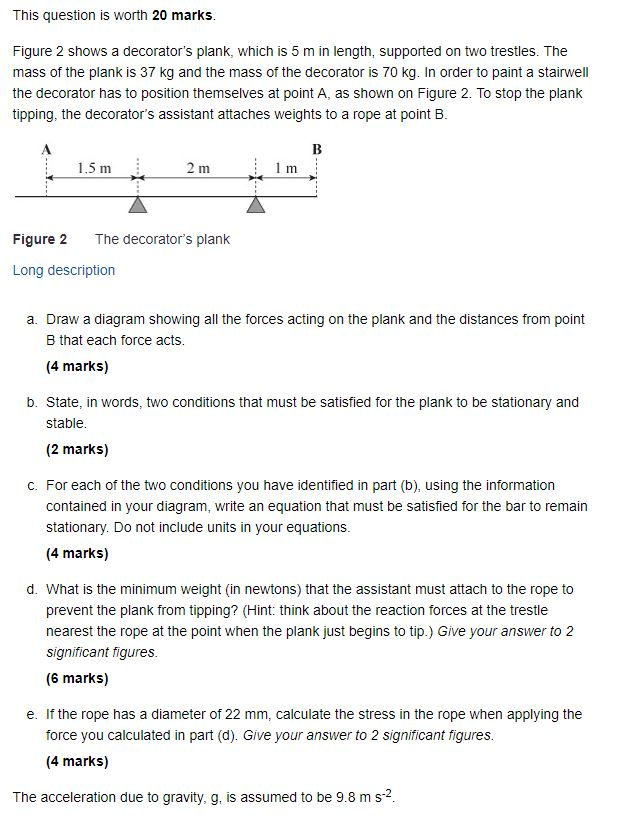This question is worth 20 marks Figure 2 shows a decorator's plank, which is 5 m in length, supported on two trestles. The mass of the plank is 37 kg and the mass of the decorator is 70 kg. In order to paint a stairwell the decorator has to position themselves at point A, as shown on Figure 2. To stop the plank tipping, the decorator's assistant attaches weights to a rope at point B Figure 2 The decorator's plank...

• ### 0.50 T. In unitve is the torque about the hinge MAGNETIC FIELDS MAG 3 An clectron...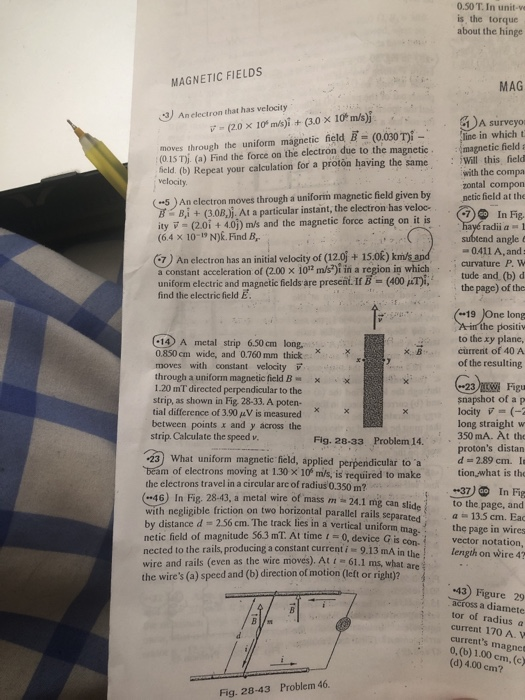0.50 T. In unitve is the torque about the hinge MAGNETIC FIELDS MAG 3 An clectron that has velocity 7- (20 x 10m/s)i + (3.0 x 10 m/s) moves through the uniform magnetic field B = (0.030 Ti - (0.15 T). (a) Find the force on the electron due to the magnetic field. (b) Repeat your calculation for a proton having the same velocity (-5) An electron moves through a uniform magnetic field given by B Bi + (3.08.). At...

Need Online Homework Help?